# PSAT Math : Monomials

## Example Questions

### Example Question #1 : How To Multiply A Monomial By A Polynomial

If you have a rectangle with a width of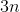and a length of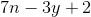, what is the area of the rectangle?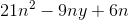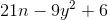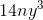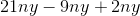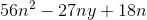Explanation:

To find the area of a rectangle, multiply the length times the width.  Therefore, you must multiplytimes.  To do that, you must multiply the monomial times each part of the trinomial, like so: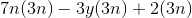### Example Question #72 : Algebra

Find the product: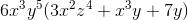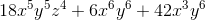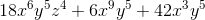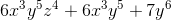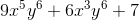Explanation:

Use the distributive property: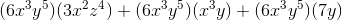Simplify: don't forget to use the rules of multiplying exponents (add them)### Example Question #1 : Monomials

Find the product: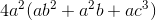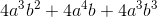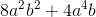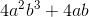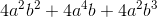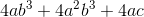Explanation:

Find the product:Use the distributive property: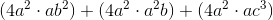When multiplying variables with exponents, add the exponents: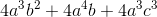### Example Question #3 : How To Multiply A Monomial By A Polynomial

Find the product: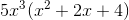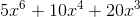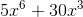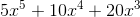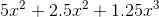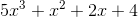Explanation:

Find the product:Use the distributive property: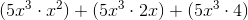### All PSAT Math Resources